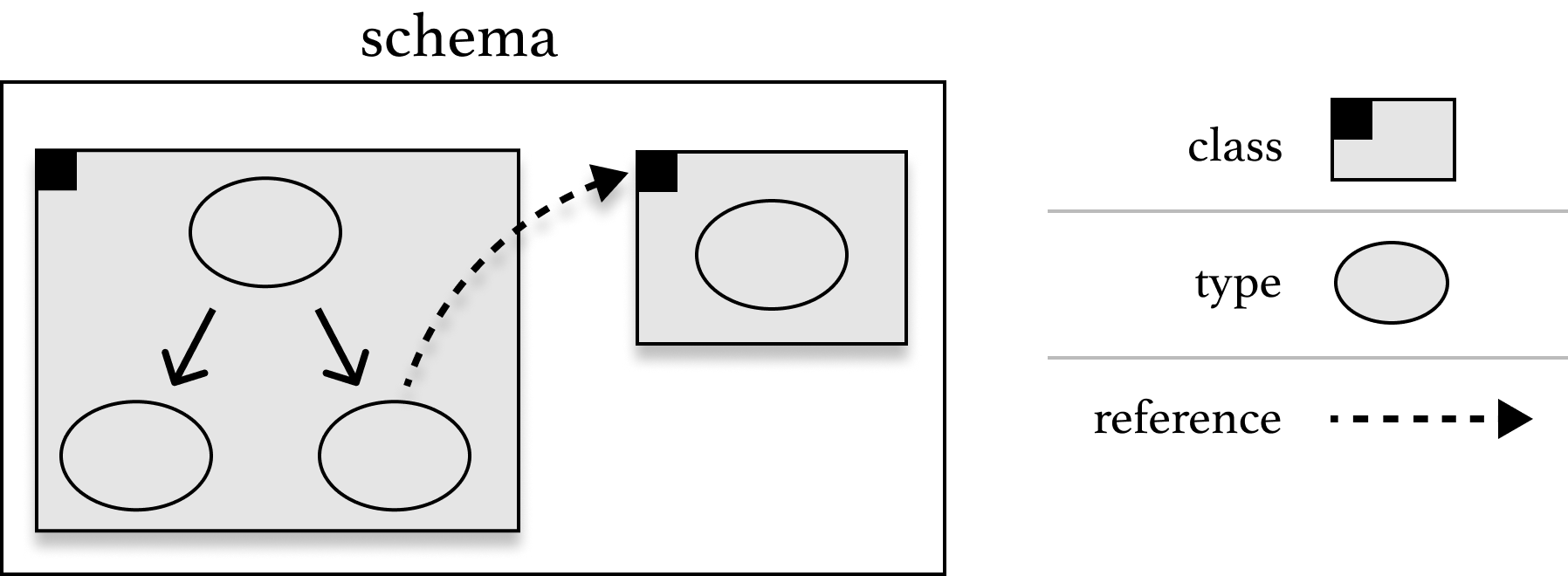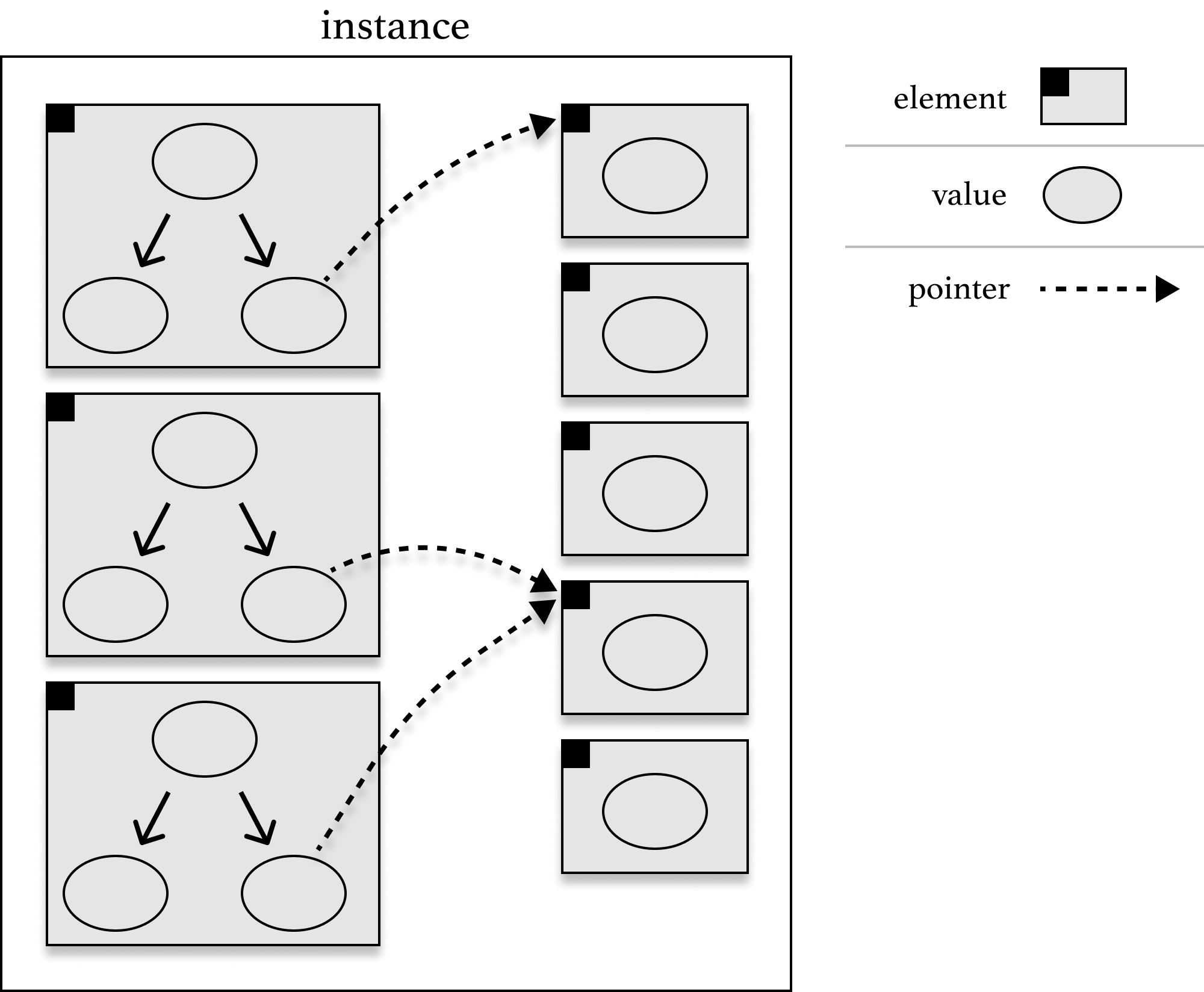# reference types

The last kind of type that we have in tasl is reference types. A reference type points to a class in the same schema. We write them with an asterisk followed by the class URI. We've already seen several of them in action:

``````namespace ex http://example.com/

class ex:Person {
ex:name -> string
ex:favoriteBook -> * ex:Book
}

class ex:Book {
ex:title -> string
ex:isbn -> <>
}``````

Here, `* ex:Book` is a reference type; we would pronounce it "a reference to `ex:Book`".

A value of a reference type is an element of the referenced class. Sometimes we'll call this value a pointer. Recall this diagram of a schema...... and this diagram of an instance:When we have a reference type that points to a given class in the same schema, its values are all pointers to specific elements (of the corresponding class) in the same instance.

## managing identity

Often you'll be faced with a choice between inlining a type and splitting it out into its own class. Let's compare the first example to this similar schema, where we just spliced the book type directly into the person class:

``````namespace ex http://example.com/

class ex:Person2 {
ex:name -> string
ex:favoriteBook -> {
ex:title -> string
ex:isbn -> <>
}
}``````

There are two major functional differences between the two schemas:

1. In the first schema (with separate classes), it's possible to have lots of books that aren't anybody's favorite. In the second example, the only way that a book can show up in an instance is as a nested value of an `ex:Person2` element.
2. In the first schema, it's easy to tell if two people have the same favorite book, since we can just ask whether the references point to the same book element. In the second schema, the best we could do is compare the values of the `ex:favoriteBook` components for different people. In this case, we're probably comfortable with comparing the values for `ex:title` and `ex:isbn`, and if they're the same for two people, concluding that they have the same favorite book. But in other cases this kind of value comparison can break down, or isn't appropriate for the actual thing you're modeling. Having separate classes allows us to have multiple elements with the exact same values.

The common theme behind both of these differences is the motto that identity is held by elements. Comparing the values (or parts of the values) of different elements is only sometimes useful.

## multi-valued properties

One common way that we use references is to model properties that can have multple values. For example, in this simple schema...

``````namespace ex http://example.com/

class ex:Person {
ex:age -> uint
ex:name -> string
}``````

... a person has to have exactly one name. We've seen how we could model a person with an optional name...

``````namespace ex http://example.com/
namespace ul http://underlay.org/ns/

class ex:Person {
ex:age -> uint
ex:name -> [
ul:none
ul:some <- string
]
}``````

... but what if we wanted to model a person with zero or more names? To do this, we have to name a separate class just for the person/name property, like this:

``````namespace ex http://example.com/

class ex:Person {
ex:age -> uint
}

class ex:Person/name {
ex:person -> * ex:Person
ex:name -> string
}``````

In this schema, we can have arbitrarily many people elements, each of whom has exactly one unsigned integer age. Separately, we have arbitrarly many `ex:Person/name`, each of which points to a person element and also has a string name. Retriving the set of names that are associated with a given person just amounts to retriving the `ex:Person/name` elements that reference to that person.

Writing separate classes for multi-properties like this is definitely a little awkward. It's good practice to give these "property classes" verbose, obvious names like `ex:Person/name` to indicate that they're not proper conceptual objects. "Property class" is an informal term for classes that are used to link other classes with values.

## unit references

Another useful general pattern is to use references to unit classes as a way to "tie things together". We've already seen this with the example of a directed graph:

``````namespace ex http://example.com/

class ex:Node {}

class ex:Edge {
ex:source -> * ex:Node
ex:target -> * ex:Node
}``````

The elements of the `ex:Node` class don't have any information associated with them; they only serve to "wire together" the graph.

But let's say we wanted to turn this schema into a hypergraph data model, where edges contain arbitrarily many nodes. Now instead of fixed `ex:source` and `ex:target` properties, we need to express that an edge can have any number of `* ex:Node` references. This is exactly the kind of multi-valued property we just saw in the last section! We can express this by making edges (in addition to nodes) a unit class and introducing a new edge-associated-with-a-node property class.

``````namespace ex http://example.com/

class ex:Node {}

class ex:Hyperedge {}

class ex:Hyperedge/includes {
ex:edge -> * ex:Hyperedge
ex:node -> * ex:Node
}``````

Multi-valued properties are also called one-to-many properties; hypergraphs are an example of modeling a many-to-many property. Each edge can have many nodes; each node can belong to many edges. But the important takeaway is that "supporting many-to-many properties" isn't built in to tasl. Instead, it's a structure that we were able to model ourselves using simple building blocks (units, products, and references).

## self-references

Technically, you can even define a class to be a reference to itself:

``````namespace ex http://example.com/

class ex:Useless * ex:Useless``````

... although this structure isn't very useful. An instance of this schema could have arbitrarly many elements of the `ex:Useless` class; each one would have to point to another (or itself).

Here's a more interesting kind of self-reference:

``````namespace ex http://example.com/

class ex:ListOfIntegers [
ul:none
ul:some <- {
ex:tail -> * ex:ListOfIntegers
}
]``````

The class `ex:ListOfIntegers` is either nothing (in the `ex:none` option) or (in the `ex:some` option) a product of two components: an `integer` and a pointer to another `ex:ListOfIntegers` element. This means that an element of `ex:ListOfIntegers` is either "null" (the empty list) or an integer and another element.

As the name implies, this effectively models a linked list of integers.

If we wanted to model non-empty linked lists, we could tweak our class declaration to express that:

``````namespace ex http://example.com/

class ex:ListOfIntegers {
... here we moved the optional operator from the top level to the `ex:tail` component. This means that now every element has to have an integer `ex:head` value, and can optionally link to another element.